interp2

Interpolation for 2-D gridded data in meshgrid format

Description

example

Vq = interp2(X,Y,V,Xq,Yq) returns interpolated values of a function of two variables at specific query points using linear interpolation. The results always pass through the original sampling of the function. X and Y contain the coordinates of the sample points. V contains the corresponding function values at each sample point. Xq and Yq contain the coordinates of the query points.

Vq = interp2(V,Xq,Yq) assumes a default grid of sample points. The default grid points cover the rectangular region, X=1:n and Y=1:m, where [m,n] = size(V). Use this syntax when you want to conserve memory and are not concerned about the absolute distances between points.

Vq = interp2(V) returns the interpolated values on a refined grid formed by dividing the interval between sample values once in each dimension.

example

Vq = interp2(V,k) returns the interpolated values on a refined grid formed by repeatedly halving the intervals k times in each dimension. This results in 2^k-1 interpolated points between sample values.

example

Vq = interp2(___,method) specifies an alternative interpolation method: 'linear', 'nearest', 'cubic', 'makima', or 'spline'. The default method is 'linear'.

example

Vq = interp2(___,method,extrapval) also specifies extrapval, a scalar value that is assigned to all queries that lie outside the domain of the sample points.

If you omit the extrapval argument for queries outside the domain of the sample points, then based on the method argument interp2 returns one of the following:

• Extrapolated values for the 'spline' and 'makima' methods

• NaN values for other interpolation methods

Examples

collapse all

Coarsely sample the peaks function.

[X,Y] = meshgrid(-3:3);
V = peaks(X,Y);

Plot the coarse sampling.

figure
surf(X,Y,V)
title('Original Sampling');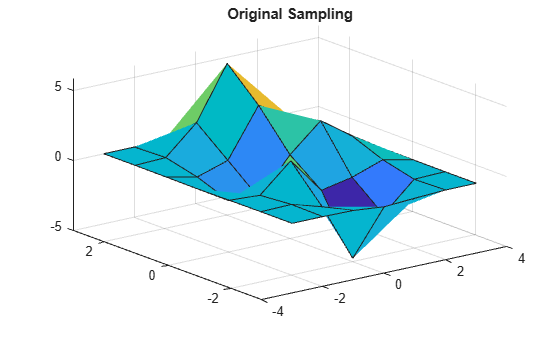Create the query grid with spacing of 0.25.

[Xq,Yq] = meshgrid(-3:0.25:3);

Interpolate at the query points.

Vq = interp2(X,Y,V,Xq,Yq);

Plot the result.

figure
surf(Xq,Yq,Vq);
title('Linear Interpolation Using Finer Grid');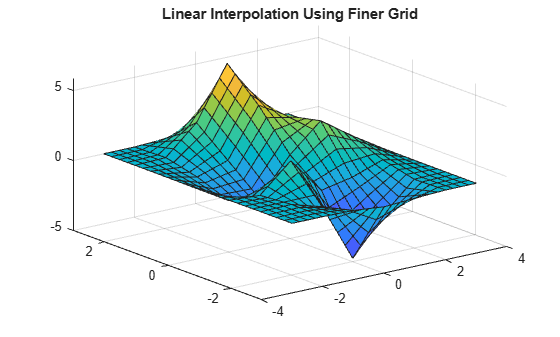Coarsely sample the peaks function.

[X,Y] = meshgrid(-3:3);
V = peaks(7);

Plot the coarse sampling.

figure
surf(X,Y,V)
title('Original Sampling');Create the query grid with spacing of 0.25.

[Xq,Yq] = meshgrid(-3:0.25:3);

Interpolate at the query points, and specify cubic interpolation.

Vq = interp2(X,Y,V,Xq,Yq,'cubic');

Plot the result.

figure
surf(Xq,Yq,Vq);
title('Cubic Interpolation Over Finer Grid');Load some image data into the workspace.

colormap gray

Isolate a small region of the image and cast it to single-precision.

V = single(X(200:300,1:25));

Display the image region.

imagesc(V);
axis off
title('Original Image')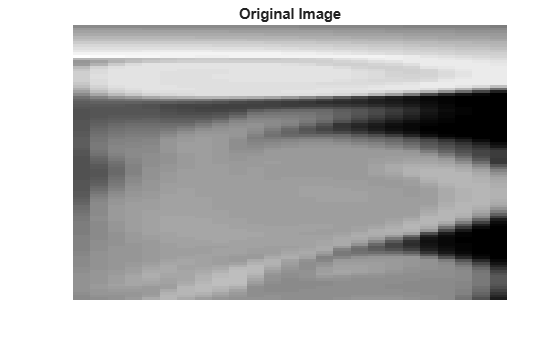Insert interpolated values by repeatedly dividing the intervals between points of the refined grid five times in each dimension.

Vq = interp2(V,5);

Display the result.

imagesc(Vq);
axis off
title('Linear Interpolation')Coarsely sample a function over the range, [-2, 2] in both dimensions.

[X,Y] = meshgrid(-2:0.75:2);
R = sqrt(X.^2 + Y.^2)+ eps;
V = sin(R)./(R);

Plot the coarse sampling.

figure
surf(X,Y,V)
xlim([-4 4])
ylim([-4 4])
title('Original Sampling')Create the query grid that extends beyond the domain of X and Y.

[Xq,Yq] = meshgrid(-3:0.2:3);

Perform cubic interpolation within the domain of X and Y, and assign all queries that fall outside to zero.

Vq = interp2(X,Y,V,Xq,Yq,'cubic',0);

Plot the result.

figure
surf(Xq,Yq,Vq)
title('Cubic Interpolation with Vq=0 Outside Domain of X and Y');Input Arguments

collapse all

Sample grid points, specified as real matrices or vectors. The sample grid points must be unique.

• If X and Y are matrices, then they contain the coordinates of a full grid (in meshgrid format). Use the meshgrid function to create the X and Y matrices together. Both matrices must be the same size.

• If X and Y are vectors, then they are treated as grid vectors. The values in both vectors must be strictly monotonic, either increasing or decreasing.

Example: [X,Y] = meshgrid(1:30,-10:10)

Data Types: single | double

Sample values, specified as a real or complex matrix. The size requirements for V depend on the size of X and Y:

• If X and Y are matrices representing a full grid (in meshgrid format), then V must be the same size as X and Y.

• If X and Y are grid vectors, then V must be a matrix containing length(Y) rows and length(X) columns.

If V contains complex numbers, then interp2 interpolates the real and imaginary parts separately.

Example: rand(10,10)

Data Types: single | double
Complex Number Support: Yes

Query points, specified as a real scalars, vectors, matrices, or arrays.

• If Xq and Yq are scalars, then they are the coordinates of a single query point.

• If Xq and Yq are vectors of different orientations, then Xq and Yq are treated as grid vectors.

• If Xq and Yq are vectors of the same size and orientation, then Xq and Yq are treated as scattered points in 2-D space.

• If Xq and Yq are matrices, then they represent either a full grid of query points (in meshgrid format) or scattered points.

• If Xq and Yq are N-D arrays, then they represent scattered points in 2-D space.

Example: [Xq,Yq] = meshgrid((1:0.1:10),(-5:0.1:0))

Data Types: single | double

Refinement factor, specified as a real, nonnegative, integer scalar. This value specifies the number of times to repeatedly divide the intervals of the refined grid in each dimension. This results in 2^k-1 interpolated points between sample values.

If k is 0, then Vq is the same as V.

interp2(V,1) is the same as interp2(V).

The following illustration shows the placement of interpolated values (in red) among nine sample values (in black) for k=2.Example: interp2(V,2)

Data Types: single | double

Interpolation method, specified as one of the options in this table.

'linear'The interpolated value at a query point is based on linear interpolation of the values at neighboring grid points in each respective dimension. This is the default interpolation method.C0
• Requires at least two grid points in each dimension

• Requires more memory than 'nearest'

'nearest'The interpolated value at a query point is the value at the nearest sample grid point. Discontinuous
• Requires two grid points in each dimension.

• Fastest computation with modest memory requirements

'cubic'The interpolated value at a query point is based on a cubic interpolation of the values at neighboring grid points in each respective dimension. The interpolation is based on a cubic convolution.C1
• Grid must have uniform spacing in each dimension, but the spacing does not have to be the same for all dimensions

• Requires at least four points in each dimension

• Requires more memory and computation time than 'linear'

'makima'Modified Akima cubic Hermite interpolation. The interpolated value at a query point is based on a piecewise function of polynomials with degree at most three evaluated using the values of neighboring grid points in each respective dimension. The Akima formula is modified to avoid overshoots.C1
• Requires at least 2 points in each dimension

• Produces fewer undulations than 'spline'

• Computation time is typically less than 'spline', but the memory requirements are similar

'spline'The interpolated value at a query point is based on a cubic interpolation of the values at neighboring grid points in each respective dimension. The interpolation is based on a cubic spline using not-a-knot end conditions.C2
• Requires four points in each dimension

• Requires more memory and computation time than 'cubic'

Function value outside domain of X and Y, specified as a real or complex scalar. interp2 returns this constant value for all points outside the domain of X and Y.

Example: 5

Example: 5+1i

Data Types: single | double
Complex Number Support: Yes

Output Arguments

collapse all

Interpolated values, returned as a real or complex scalar, vector, or matrix. The size and shape of Vq depends on the syntax you use and, in some cases, the size and value of the input arguments.

SyntaxesSpecial ConditionsSize of VqExample
interp2(X,Y,V,Xq,Yq)
interp2(V,Xq,Yq)
and variations of these syntaxes that include method or extrapval
Xq, Yq are scalarsScalarsize(Vq) = [1 1] when you pass Xq and Yq as scalars.
Same as aboveXq, Yq are vectors of the same size and orientationVector of same size and orientation as Xq and YqIf size(Xq) = [100 1]
and size(Yq) = [100 1],
then size(Vq) = [100 1].
Same as aboveXq, Yq are vectors of mixed orientationMatrix in which the number of rows is length(Yq), and the number of columns is length(Xq)If size(Xq) = [1 100]
and size(Yq) = [50 1],
then size(Vq) = [50 100].
Same as aboveXq, Yq are matrices or arrays of the same sizeMatrix or array of the same size as Xq and YqIf size(Xq) = [50 25]
and size(Yq) = [50 25],
then size(Vq) = [50 25].
interp2(V,k)
and variations of this syntax that include method or extrapval
None

Matrix in which the number of rows is:
2^k * (size(V,1)-1)+1,

and the number of columns is:
2^k * (size(V,2)-1)+1

If size(V) = [10 20]
and k = 2,
then size(Vq) = [37 77].

collapse all

Strictly Monotonic

A set of values that are always increasing or decreasing, without reversals. For example, the sequence, a = [2 4 6 8] is strictly monotonic and increasing. The sequence, b = [2 4 4 6 8] is not strictly monotonic because there is no change in value between b(2) and b(3). The sequence, c = [2 4 6 8 6] contains a reversal between c(4) and c(5), so it is not monotonic at all.

Full Grid (in meshgrid Format)

For interp2, the full grid is a pair of matrices whose elements represent a grid of points over a rectangular region. One matrix contains the x-coordinates, and the other matrix contains the y-coordinates. The values in the x-matrix are strictly monotonic and increasing along the rows. The values along its columns are constant. The values in the y-matrix are strictly monotonic and increasing along the columns. The values along its rows are constant. Use the meshgrid function to create a full grid that you can pass to interp2.

For example, the following code creates a full grid for the region, –1 ≤ x ≤ 3 and 1 ≤ y ≤ 4:

[X,Y] = meshgrid(-1:3,(1:4))
X =

-1     0     1     2     3
-1     0     1     2     3
-1     0     1     2     3
-1     0     1     2     3

Y =

1     1     1     1     1
2     2     2     2     2
3     3     3     3     3
4     4     4     4     4

Grid vectors are a more compact format to represent a grid than the full grid. The relation between the two formats and the matrix of sample values V is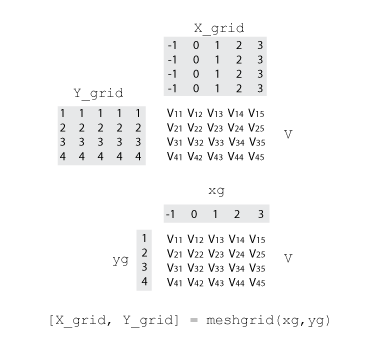Grid Vectors

For interp2, grid vectors consist of a pair of vectors that define the x- and y-coordinates in a grid. The row vector defines x-coordinates, and the column vector defines y-coordinates.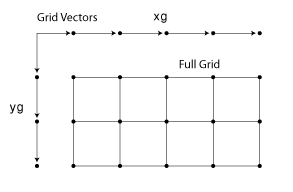For example, the following code creates the grid vectors that specify the region, –1 ≤ x ≤ 3 and 1 ≤ y ≤ 4:

x = -1:3;
y = (1:4)';

Scattered Points

For interp2, scattered points consist of a pair of arrays that define a collection of points scattered in 2-D space. One array contains the x-coordinates, and the other contains the y-coordinates.

For example, the following code specifies the points, (2,7), (5,3), (4,1), and (10,9):

x = [2 5; 4 10];
y = [7 3; 1 9];